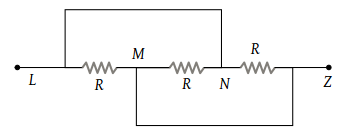# NEET Questions Solved

Three equal resistances each of value R are joined as shown in the figure. The equivalent resistance between M and N is(1) R

(2) 2R

(3) $\frac{R}{2}$

(4) $\frac{R}{3}$

(4) Three resistances are in parallel.

$\frac{1}{R\text{'}}=\frac{1}{R}+\frac{1}{R}+\frac{1}{R}=\frac{3}{R}$

The equivalent resistance $R\text{'}=\frac{R}{3}\Omega$

Difficulty Level:

• 20%
• 10%
• 11%
• 62%